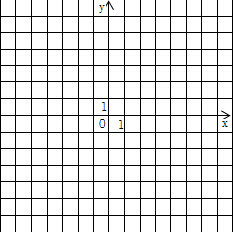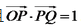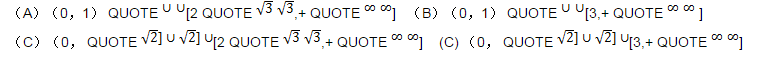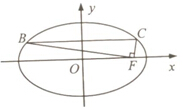(I)在答题卡第(24)题图中画出y=f(x)的图像

(II)求不等式丨f(x)丨>1的解集• 设O为坐标原点,动点M在椭圆C:x平方/2+y平方=1上,过M做x轴的垂线,垂足为N,点P满足

设O为坐标原点，动点M在椭圆C:x平方/2+y平方=1上，过M做x轴的垂线，垂足为N，点P满足(1) 求点P的轨迹方程;

(2) 设点Q在直线x=-3上，且证明：过点P且垂直于OQ的直线l过C的左焦点F.

• 已知当x∈[0,1]时,函数y=(mx-1)2 的图象与y= QUOTE √ x√ x+m的图象有且只有一个交点,则正实数m的取值范围是

已知当x∈[0,1]时，函数y=(mx-1)2 的图象与y= QUOTE √ x√ x+m的图象有且只有一个交点，则正实数m的取值范围是• 如图,在平面直角坐标系xOy中,F是椭圆x2/a2+y2/b2=1(a>b>0)的右焦点,直线y=b/2与椭圆交

如图，在平面直角坐标系xOy中，F是椭圆x2/a2+y2/b2=1(a>b>0)的右焦点，直线y=b/2与椭圆交于B，C两点，且∠BFC=90°,则该椭圆的离心率是_____.• 在?ABC中,角A,B,C所对的边分别为a,b,c,∠ABC=120o,∠ABC的平分线交AC于点D,且BD=1,则4a+c的最小值

在?ABC中，角A,B,C所对的边分别为a,b,c，∠ABC=120o，∠ABC的平分线交AC于点D，且BD=1，则4a+c的最小值为_______．

• (本小题满分14分) 已知a,β为锐角,tana=4/3,cos(a+β)=-√5/5.(1)求cos2a的值; (2)求tan(a-β)的值

（本小题满分14分） 已知a,β为锐角，tana=4/3，cos(a+β)=-√5/5．

（1）求cos2a的值； （2）求tan(a-β)的值．

• 若函数f(x)=2x^3-ax^2+1(a∈R)在(0,+∞)内有且只有一个零点,则f(x)在[-1,1]上的最大值与最小值的和

若函数f(x)=2x^3-ax^2+1(a∈R)在(0,+∞)内有且只有一个零点，则f(x)在[-1,1]上的最大值与最小值的和为_______．该问题答案仅对会员开放，欢迎开通会员

1个月
¥39.8
1.28元/天
3个月
¥49.8
0.54元/天
1周
¥29.8
4.26元/天微信支付支付宝支付### 请使用微信扫码支付(元)